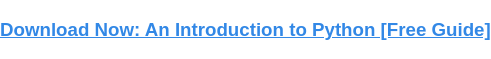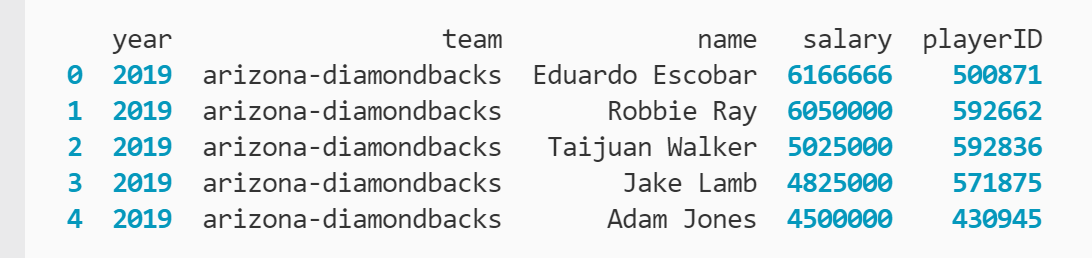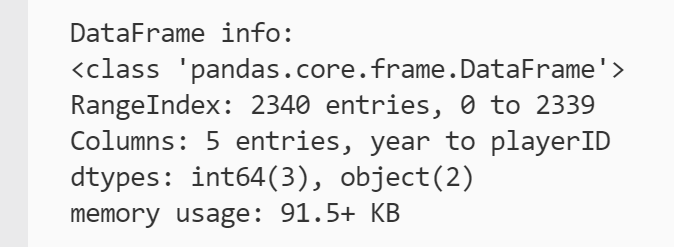# How to Find Pandas DataFrame Size, Shape, and Dimensions PropertiesUpdated:

Published:

pandas is a Python library that excels at capturing and manipulating relational data. A central focus of pandas is data analysis and generating new insights about the values stored in DataFrames and Series. Achieving a high-level view of the data can also provide a better understanding of the attributes of your data set.Pandas has built-in properties that offer metrics on the size, shape, and dimensions of your DataFrames. These attributes can inform your analysis.

For example, you may find that your DataFrame has less than a hundred cells, so a shortcut that isn't as efficient in execution could be acceptable versus a more efficient but harder to implement function. However, if the DataFrame has thousands of cells, then it will be worthwhile to strategically select the functions you use to manipulate and analyze your data.

Let's examine how to access these attributes.

The following tutorials use the Major League Baseball (MLB) Players Salaries data set available from Kaggle. You can download the CSV file if you'd like to follow along with the examples.

To start, you import the pandas library and use the .read_csv() method to convert the data set into a DataFrame, which is assigned to the variable baseball_df:

``` ```
import pandas as pd
``````

You can confirm the DataFrame was created by using the .head() method, which provides a preview by pulling the first five rows of the DataFrame:

``` ```
``````

The outcome of printing the .head() call is below.You can see five rows of data on MLB players' salaries organized across five columns. In other words, you have a DataFrame. With large data sets, it's more efficient to call a preview method like .head for quick confirmations like this than to try to print the entire DataFrame.

Now that you created the DataFrame, you can start to find its attributes. Let's start with the total number of cells:

``` ```
print(baseball_df.size)
``````

The output of the print statement is below.You can see that your DataFrame has 11,700 cells. In other words, you have 11,700 values in your data set.

You can also save this value to a variable for future reference and calculations:

``` ```
df_size = baseball_df.size
``````

Given the current examination of size, you may wonder if there's a limit to how large a DataFrame can be. Let's address this common question next.

### Is there a size limit for Pandas DataFrames?

The short answer is yes, there is a size limit for pandas DataFrames, but it's so large you will likely never have to worry about it.

The long answer is the size limit for pandas DataFrames is 100 gigabytes (GB) of memory instead of a set number of cells. In effect, this benchmark is so large that it would take an extraordinarily large data set to reach it.

To provide some context, you now know that the baseball_df DataFrame holds 11,700 values. To see what this translates to in memory, you can use the .info() method:

``` ```
baseball_df.info(verbose = False)
``````

The printout is below.With 11,700 values, baseball_df hasn't even cracked 100 kilobytes yet. That's less than one-tenth of a megabyte or one-thousandth of a GB. By doing some rudimentary conversions, it appears that you would need more than 12 billion data cells to even approach the size limit based on the current data set.

Now that you better understand the size of your DataFrame and its size constraints (or lack of), let's dive into how to find the DataFrame structure.

## How to Find the Shape of a Pandas DataFrame

Size alone doesn't reveal everything about your DataFrame. Another common attribute is the shape of a DataFrame.

The workflow for the .shape property is similar to the .size example:

``` ```
print(baseball_df.shape)
``````

The result of the print statement is below.Here, the .shape property returns a tuple showing the DataFrame has 2,340 rows and 5 columns. A tuple is similar to a Python list in many respects; the biggest difference is that tuples are immutable, meaning they cannot be changed once declared.

Like lists, you can access each of the values at their corresponding index value:

``` ```
baseball_df.shape
# equals 2340
baseball_df.shape
# equals 5
``````

Using indexing, you can extract each of these values into variables to store and use in calculations. You can also use a Python shorthand known as unpacking to declare both variables on the same line:

``` ```
row_count, column_count = baseball_df.shape
``````

Let's examine the final attribute: dimensions.

## How to Fetch the Dimensions of a Pandas DataFrame

Like the other two properties, accessing the dimensions of a pandas DataFrame is straightforward. Just use .ndim:

``` ```
print(baseball_df.ndim)
``````

The result of the print statement is below.Here, you can see that the .ndim property returns an integer 2. This matches expectations because DataFrames are two-dimensional data structures, meaning they have rows and columns. If .ndim returned 1, then the baseball_df variable is a pandas Series, which is a one-dimensional data structure.

## Pandas DataFrame size, shape, and dimensions power strategic analysis.

Optimized data analysis is a key benefit of the pandas library. This includes not only insights derived from the values themselves but metadata for the data set as a whole. Pandas offers easy shortcuts to pull key attributes about DataFrames' size, shape, and dimensions, providing a quick start to your metadata collection so you can find the answers you need faster.Topics: What Is Python?

## Related Articles

We're committed to your privacy. HubSpot uses the information you provide to us to contact you about our relevant content, products, and services. You may unsubscribe from these communications at any time. For more information, check out our Privacy Policy.

A guide for marketers, developers, and data analysts.